# Reproduce the results of Repeated Measures Correlation paper

### 1. Figure 1: rmcorr and reg plot

# echo = FALSE, warning = FALSE, results =  "hide",
set.seed(1)

initX <- rnorm(50)
newY <- NULL
newX <- NULL
sub <- rep(1:10, each = 5)

rsq <- .9

for (i in 1:10){
tempData <- initX[sub == i] + addx
sdx <- sd(tempData)
sdnoise <- sdx * (sqrt((1-rsq)/rsq))
tempy <- tempData + rnorm(5,0,sdnoise) + rnorm(1,0,3)
newY <- c(newY, tempy)
newX <- c(newX,tempData)
}

exampleMat <-data.frame(cbind(sub,newX,newY))

###standard averaged regression plot
submeanx <- aggregate(exampleMat$newX, by = list(exampleMat$sub), mean)
submeany <- aggregate(exampleMat$newY, by = list(exampleMat$sub), mean)
mypal <- colorRampPalette(RColorBrewer::brewer.pal(10,'Paired'))
cols <- mypal(10)

example.rmc <- rmcorr(sub,newX,newY,exampleMat)
#> Warning in rmcorr(sub, newX, newY, exampleMat): 'sub' coerced into a factor

#for graphing: get the rmcorr coefficient (rounded) and p-value (using pvals.fct)
example.rmc.r <- sprintf("%.2f", round(example.rmc$r, 2)) example.rmc.p <- pvals.fct(example.rmc$p)

#ditto for cor
stdr <- cor.test(submeanx[,2], submeany[,2])
example.cor.r <- sprintf("%.2f", round(stdr$estimate, 2)) example.cor.p <- pvals.fct(stdr$p.value)

par(mfrow = c(1, 2), mgp = c(2.5, .75, 0), mar = c(4,4,2,1), cex = 1.2)

plot(example.rmc, xlab = "x", ylab = "y",
overall = F, palette = mypal, las = 1, ylim = c(-6, 6.5))
title("A)", adj = 0) #Removed for Frontiers formatting

text(1.25, -5, adj = 1, bquote(italic(r[rm])~"="~ .(example.rmc.r)))
text(1.25, -5.75, adj = 1, bquote(italic('p')~.(example.rmc.p)))

plot(submeanx[,2], submeany[,2], pch = 16, col = cols, las = 1,
xlab = "x (averaged for each participant)",
ylab = "y (averaged for each participant)", ylim=c(-6,6.5), xlim=c(-3, 1))

text(0.90, -5, adj = 1, bquote(italic('r')~"="~ .(example.cor.r)))
text(0.90, -5.75, adj = 1, bquote(italic('p')~"="~.(example.cor.p)))

abline(lm(submeany[,2]~submeanx[,2]),col="gray50")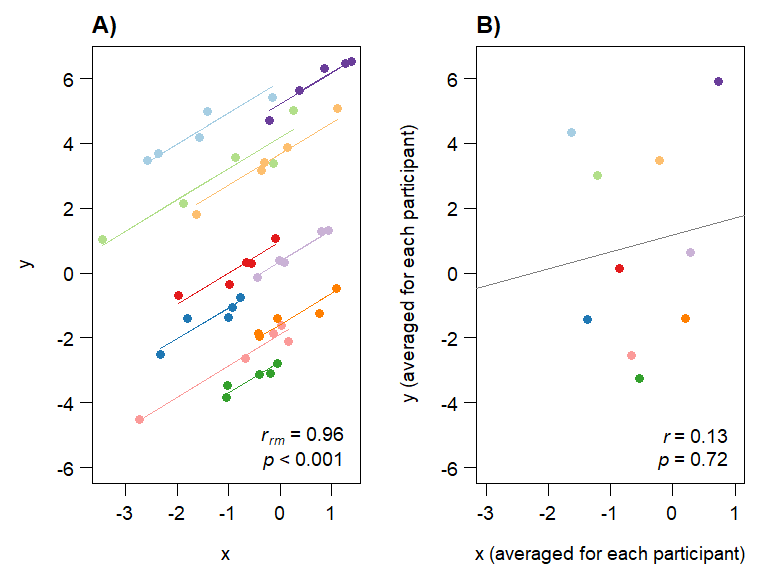#(A) Rmcorr plot: rmcorr plot for a set of hypothetical data and (B) simple regression plot: the
#corresponding regression plot for the same data averaged by participant.

#dev.copy2eps(file="plots/Figure1_Rmcorr_vs_reg.eps", height = 6, width = 8)
#dev.copy(pdf, file="plots/Figure1_Rmcorr_vs_reg.pdf", height = 6, width = 8)
#dev.off()

### 2. Figure 2: rmcorr vs OLS reg

par(mfrow = c(3,3), mar = c(1,1,.5,.5), mgp = c(2.5,.75,0),
oma = c(4,4,4,0), cex = 1.1)

makeminiplot <- function(subxs, sub.slope, intercept, constant=0, xax = "n",
yax = "n", legend = F){

mypal <- colorRampPalette(RColorBrewer::brewer.pal(10,'Paired'))
cols <- mypal(3)

# cols <- c("#A6CEE3", "#9D686D", "#6A3D9A")

subys <- list(3)
for (i in 1:3){
subys[[i]] <- subxs[[i]] * sub.slope + intercept*i + constant
}

plot(subxs[],subys[], type = "n", xlim =c(0,4), ylim = c(0,10),
xlab = "", ylab = "", xaxt = xax, yaxt = yax, las = 1)

allx <- unlist(subxs)
ally <- unlist(subys)
abline(lm(ally~allx))

for (i in 1:3) {
lines(subxs[[i]],subys[[i]], type = "o", col = cols[i], pch = 16)
}

if (legend) legend('bottomright', legend = "OLS", lwd = 1.25, bty = "n",
cex = 1, inset = -0.03)
}

subxs <- list(3)
subxs[] <- seq(0,2,.25)
subxs[] <- seq(1,3,.25)
subxs[] <- seq(2,4,.25)

#ols is positive
makeminiplot(subxs, -1, 4, yax = "s", legend = T)
makeminiplot(subxs, 0, 2.75)
makeminiplot(subxs, 1, 1.5)

#ols is flat
makeminiplot(subxs, -1.5, 2.45, 3, yax = "s")
makeminiplot(subxs, 0, 0, 5)
makeminiplot(subxs, 1.5, -2.4, 7)

#ols is negative
makeminiplot(subxs, -.75, -2, 10, yax = "s", xax = "s")
makeminiplot(subxs, 0, -3.1, 10.9, xax = "s")
makeminiplot(subxs, .9, -4.6, 12, xax = "s")

mtext(side = 1, outer = T, line = 1.5, "x", at = c(.175, .5, .85))
mtext(side = 2, outer = T, line = 1.5, "y", at = c(.175, .5, .85), las = 1)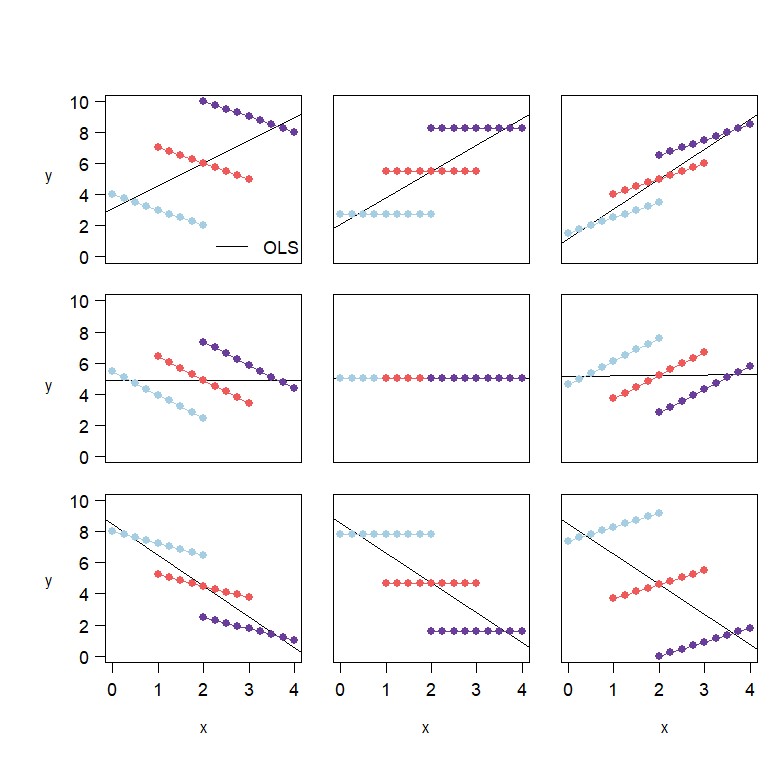# mtext(side = 3, outer = T, line = .5,
#       c("a) rmcorr = -1", "b) rmcorr = 0", "c) rmcorr = 1"),
#       at = c(.175, .5, .85), las = 1, cex = 1.5)

#Figure 2. These notional plots illustrate the range of potential similarities and differences
#in the intra-individual association assessed by rmcorr and the inter-individual association
#assessed by ordinary least squares (OLS) regression. Rmcorr-values depend only on the
#intra-individual association between variables and will be the same across different patterns
#of inter-individual variability. (A) rrm = −1: depicts notional data with a perfect negative
#intra-individual association between variables, (B) rrm = 0: depicts data with no
#intra-individual association, and (C) rrm = 1: depicts data with a perfect positive
#intra-individual association. In each column, the relationship between subjects
#(inter-individual variability) is different, which does not change the rmcorr-values within a
#column. However, this does change the association that would be predicted by OLS regression
#(black lines) if the data were treated as IID or averaged by participant.

#dev.copy2eps(file="plots/Figure2_Rmcorr_vs_OLS.eps", height = 8, width = 8)
#dev.copy(pdf, file="plots/Figure2_Rmcorr_vs_OLS.pdf", height = 8, width = 8)
#dev.off()

### 3. Figure 3: rmcorr w/data transformations

set.seed(10)
initX <- rnorm(15)
newY <- NULL
newX <- NULL
sub <- rep(1:3, each = 5)
rsq <- .7
for (i in 1:3){

tempData <- initX[sub == i] + addx
sdx <- sd(tempData)
sdnoise <- sdx * (sqrt((1-rsq)/rsq))
tempy <- tempData + rnorm(5,0,sdnoise) + rnorm(1,addy,1)
newY <- c(newY, tempy)
newX <- c(newX,tempData)
}

par(mfrow=c(1,3), mar = c(4,4,2,2), mgp = c(2.75, .75, 0), cex = 1.2)

###original plot
exampleMat <-data.frame(cbind(sub,newX,newY))
example1.rmc <- rmcorr(sub,newX,newY,exampleMat)
#> Warning in rmcorr(sub, newX, newY, exampleMat): 'sub' coerced into a factor

mypal <- colorRampPalette(RColorBrewer::brewer.pal(10,'Paired'))

plot(example1.rmc, xlab = "x", ylab = "",
overall = F, palette = mypal, xlim = c(-3.5, 1), ylim = c(-2.5,2), las = 1)

example1.rmc.r <- sprintf("%.2f", round(example1.rmc$r, 2)) example1.rmc.p <- pvals.fct(example1.rmc$p)

text(-3.5, 2, adj = 0, bquote(italic(r[rm])~"="~ .(example1.rmc.r)))
text(-3.5, 1.75, adj = 0, bquote(italic('p')~.(example1.rmc.p)))
mtext(side = 2, "y", las = 1, line = 2.5, cex = 1.2)

###add 1 to all x's, multiply by 2
exampleMat2 <- exampleMat
exampleMat2$newX <- exampleMat2$newX * .5 + 1
example2.rmc <- rmcorr(sub, newX, newY, exampleMat2)
#> Warning in rmcorr(sub, newX, newY, exampleMat2): 'sub' coerced into a factor

example2.rmc.r <- sprintf("%.2f", round(example2.rmc$r, 2)) example2.rmc.p <- pvals.fct(example2.rmc$p)

plot(example2.rmc, xlab = "x", ylab = "", overall = F,
palette = mypal, xlim = c(-3.5, 1), ylim = c(-2.5,2), las = 1)

text(-3.5, 2, adj = 0, bquote(italic(r[rm])~"="~ .(example2.rmc.r)))
text(-3.5, 1.75, adj = 0, bquote(italic('p')~.(example2.rmc.p)))

mtext(side = 2, "y", las = 1, line = 2.5, cex = 1.2)

###just add -2 to sub3's ys
exampleMat3 <- exampleMat
exampleMat3$newY[11:15] <- exampleMat3$newY[11:15] - 2
example3.rmc <- rmcorr(sub, newX, newY, exampleMat3)
#> Warning in rmcorr(sub, newX, newY, exampleMat3): 'sub' coerced into a factor

example3.rmc.r <- sprintf("%.2f", round(example3.rmc$r, 2)) example3.rmc.p <- pvals.fct(example3.rmc$p)

plot(example3.rmc, xlab = "x", ylab = "", overall = F,
palette = mypal, xlim = c(-3.5, 1), ylim = c(-2.5,2), las = 1)

text(-3.5, 2, adj = 0, bquote(italic(r[rm])~"="~ .(example3.rmc.r)))
text(-3.5, 1.75, adj = 0, bquote(italic('p')~.(example3.rmc.p)))

mtext(side = 2, "y", las = 1, line = 2.5, cex = 1.2)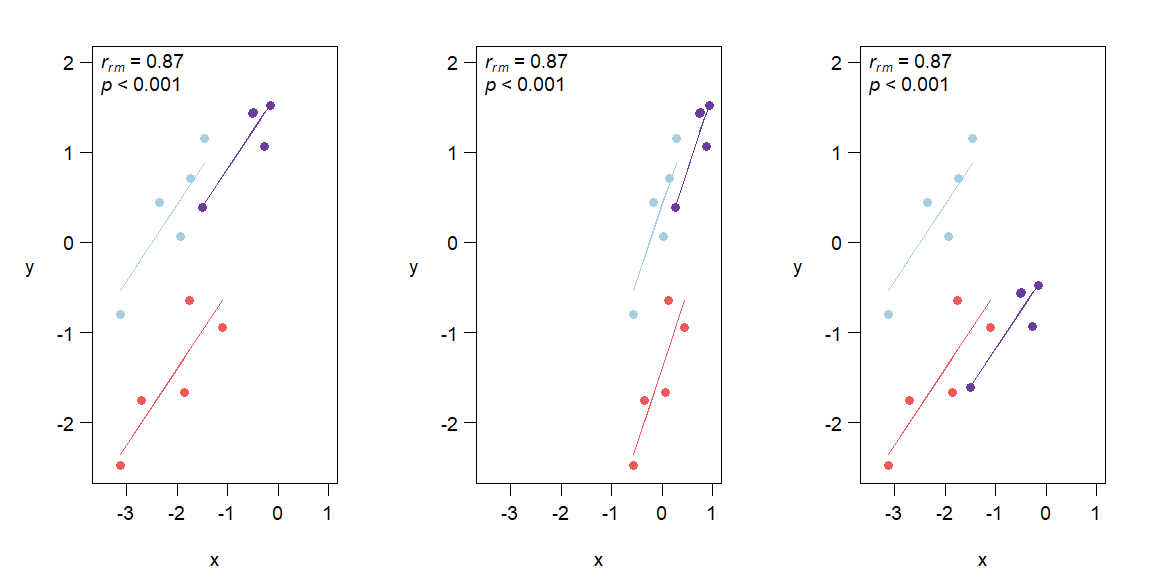#Figure 3. Rmcorr-values (and corresponding p-values) do not change with linear
#transformations of the data, illustrated here with three examples: (A) original, (B) x/2 + 1, and (C) y − 1.

#dev.copy2eps(file="plots/Figure3_Transformations.eps", height = 6, width = 12)
#dev.copy(pdf, file="plots/Figure3_Transformations.pdf", height = 6, width = 12)
#dev.off()

### 4. Figure 4: Power curves

power.rmcorr<-function(k, N, effectsizer, sig)
{
pwr.r.test(n = ((N)*(k-1))+1, r = effectsizer, sig.level = sig)
#df are specified this way because pwr.r.test assumes the input is N, so it uses N - 2 for the df
}

par(mfrow=c(1,3), cex.lab=1.50, cex.axis=1.40, cex.sub=1.40, mar=c(4.5,4.5,1.75,1))

#Small effect size
k<-c(3, 5, 10, 20)
nvals <- seq(6, 300)
powPearsonSmall <- sapply(nvals, function (x) pwr.r.test(n=x, r=0.1)$power) bluecolors<-c("#c6dbef", "#9ecae1", "#6baed6", "#4292c6", "#2171b5", "#084594") plot(nvals, seq(0,1, length.out=length(nvals)), xlab=expression(Sample~Size~"("*italic('N')*")"), yaxt = "n", ylab = "Power", las = 1, col = "white", xlim=c(0,300)) axis(1, at = seq(0, 300, 100)) yLabels <- seq(0, 1, 0.2) axis(2, at=yLabels, labels=sprintf(round(100*yLabels), fmt="%2.0f%%"), las=1, cex.sub = 2) for (i in 1:4) { powvals <- sapply(nvals, function (x) power.rmcorr(k[i], x, 0.1, 0.05)$power)
lines(nvals, powvals, lwd=2.5, col=bluecolors[i+1])
}
legend("bottomright", lwd=2.5, col=bluecolors, bty= 'n', legend=c("1", "3", "5", "10", "20"), title = expression(italic('k')),
cex = 1.2)
lines(nvals, powPearsonSmall, col=bluecolors, lwd= 2.5)
abline(a = 0.8, b=0, col=1, lty=2, lwd= 2.5)

#Medium effect size
k<-c(3, 5, 10, 20)
nvals <- seq(6, 60)
powPearsonMedium <- sapply(nvals, function (x) pwr.r.test(n=x, r=0.3)$power) greencolors<-c("#c7e9c0","#a1d99b","#74c476","#41ab5d","#238b45","#005a32") #orangecols<-brewer.pal(9, "Oranges") #orangecols3<-c(orangecols,orangecols,orangecols,orangecols,orangecols) plot(nvals, seq(0,1, length.out=length(nvals)), xlab=expression(Sample~Size~"("*italic('N')*")"), yaxt = "n", ylab = "Power", las = 1, col = "white", xlim=c(0,60)) axis(1, at = seq(0, 60, 20)) yLabels <- seq(0, 1, 0.2) axis(2, at=yLabels, main = "Power", labels=sprintf(round(100*yLabels), fmt="%2.0f%%"), las=1) for (i in 1:4) { powvals <- sapply(nvals, function (x) power.rmcorr(k[i], x, 0.3, 0.05)$power)
lines(nvals, powvals, lwd=2.5, col=greencolors[i+1])
}
legend("bottomright", lwd=2, col=greencolors, bty = 'n', legend=c("1", "3", "5", "10", "20"), title = expression(italic('k')),
cex = 1.2)
lines(nvals, powPearsonMedium, col=greencolors, lwd = 2.5)
abline(a = 0.8, b=0, col=1, lty=2, lwd= 2.5)

#Large effect size
k<-c(3, 5, 10, 20)
nvals <- seq(6, 30)
powPearsonlarge <- sapply(nvals, function (x) pwr.r.test(n=x, r=0.5)$power) purplecolors<-c("#f2f0f7", "#dadaeb", "#bcbddc", "#9e9ac8", "#807dba", "#6a51a3", "#4a1486") plot(nvals, seq(0,1, length.out=length(nvals)), xlab=expression(Sample~Size~"("*italic('N')*")"), yaxt = "n", ylab = "Power", las = 1, col = "white", xlim=c(0,30)) axis(1, at = seq(0, 40, 10)) yLabels <- seq(0, 1, 0.2) axis(2, at=yLabels, main = "Power", labels=sprintf(round(100*yLabels), fmt="%2.0f%%"), las=1) for (i in 1:4) { powvals <- sapply(nvals, function (x) power.rmcorr(k[i], x, 0.5, 0.05)$power)
lines(nvals, powvals, lwd=2.5, col=purplecolors[i+2])
}
legend("bottomright", lwd=2, col=purplecolors, legend=c("1", "3", "5", "10", "20"), bty = 'n', title = expression(italic('k')),
cex = 1.2)
abline(a = 0.8, b=0, col=1, lty=2, lwd= 2.5)
lines(nvals, powPearsonlarge, col=purplecolors, lwd = 2.5)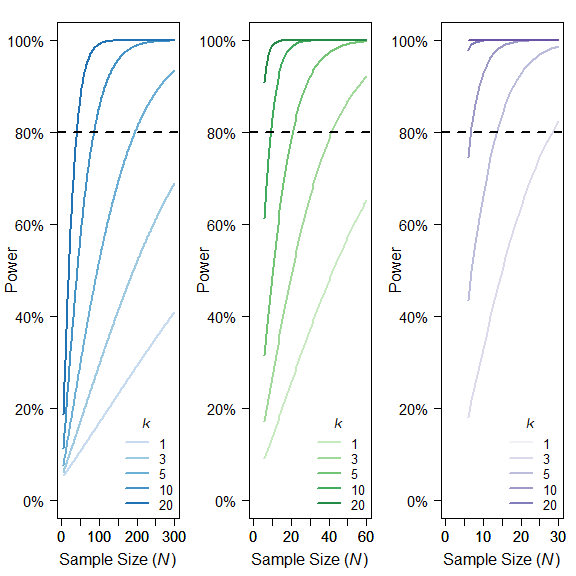#Figure 4. Power curves for (A) small, rrm, and r = 0.10, (B) medium, rrm, and r = 0.3, and (C) large effect sizes, rrm,
#and r = 0.50. X-axis is sample size. Note the sample size range differs among the panels. Y-axis is power. k denotes
#the number of repeated paired measures. Eighty percent power is indicated by the dotted black line. For rmcorr, the power of
#k = 2 is asymptotically equivalent to k = 1. A comparison to the power for a Pearson correlation with one data point per
#participant (k = 1) is also shown.

#dev.copy2eps(file="plots/Figure4_Power_curves.eps", height = 6, width = 6)
#dev.copy(pdf, file="plots/Figure4_Power_curve.pdf", height = 6, width = 6)
#dev.off()

### 5. Brain volume and age rmcorr and simple reg/cor results and Figure 5

#### rmcorr and simple reg results

#Note for details on Raz: Data captured from Figure 8, Cerebellar Hemispheres (lower right)
#a) Reproduce correlations in the paper: Cross-sectional (correlation at Time 1)
Time1raz2005<-subset(raz2005, Time == 1)
Time2raz2005<-subset(raz2005, Time == 2)
a1.rtest <- cor.test(Time1raz2005$Age, Time1raz2005$Volume)
a2.rtest <- cor.test(Time2raz2005$Age, Time2raz2005$Volume)

a1.lm <- lm(Time1raz2005$Volume ~ Time1raz2005$Age)
a2.lm <- lm(Time2raz2005$Volume ~ Time2raz2005$Age)

summary.a1.lm <- summary(a1.lm)
summary.a2.lm <- summary(a2.lm)

a1.lm.r <- sprintf("%.2f", round(a1.rtest$estimate, 2)) #Same as Pearson correlation for simple regression a1.lm.p <- pvals.fct(summary.a1.lm$coefficients[2,4])

a2.lm.r <- sprintf("%.2f", round(a2.rtest$estimate ,2)) #Same as Pearson correlation for simple regression a2.lm.p <- pvals.fct(summary.a2.lm$coefficients[2,4])

#b) rmcorr analysis
brainvolage.rmc <- rmcorr(participant = Participant, measure1 = Age, measure2 = Volume, dataset = raz2005)
#> Warning in rmcorr(participant = Participant, measure1 = Age, measure2 = Volume, :
#> 'Participant' coerced into a factor
print(brainvolage.rmc)
#>
#> Repeated measures correlation
#>
#> r
#> -0.7044077
#>
#> degrees of freedom
#> 71
#>
#> p-value
#> 3.561007e-12
#>
#> 95% confidence interval
#> -0.8053581 -0.5637514

rmcorr.5b.r <- sprintf("%.2f", round(brainvolage.rmc$r, 2)) rmcorr.5b.p <- pvals.fct(brainvolage.rmc$p)

#c) simple regression on averaged data

avgRaz2005 <- aggregate(raz2005[,3:4], by = list(raz2005$Participant), mean) avg.lm <- lm(Volume~Age, data = avgRaz2005) summary.av.lm <- summary(avg.lm) c.rtest <- cor.test(avgRaz2005$Age, avgRaz2005$Volume) print(c.rtest) #> #> Pearson's product-moment correlation #> #> data: avgRaz2005$Age and avgRaz2005$Volume #> t = -3.4912, df = 70, p-value = 0.000837 #> alternative hypothesis: true correlation is not equal to 0 #> 95 percent confidence interval: #> -0.5662456 -0.1684542 #> sample estimates: #> cor #> -0.3850943 fig.5c.r <- sprintf("%.2f", round(c.rtest$estimate,2))
fig.5c.p <-  pvals.fct(summary.av.lm$coefficients[2,4]) #Not graphed in Figure 5 #d) simple regression on aggregated data (incorrect overfit model): #Although in this case it doesn't matter brainvolage.lm<-lm(Volume~Age, data = raz2005) print(brainvolage.lm) #> #> Call: #> lm(formula = Volume ~ Age, data = raz2005) #> #> Coefficients: #> (Intercept) Age #> 151.9068 -0.3399 d.rtest <- cor.test(raz2005$Age, raz2005$Volume) print(d.rtest) #> #> Pearson's product-moment correlation #> #> data: raz2005$Age and raz2005$Volume #> t = -5.165, df = 142, p-value = 7.984e-07 #> alternative hypothesis: true correlation is not equal to 0 #> 95 percent confidence interval: #> -0.5269809 -0.2503991 #> sample estimates: #> cor #> -0.3976861 layout(matrix(c(1,3,4,2,3,4), 2, 3, byrow = T)) #a par(mar = c(1,4,4,2), oma = c(0,2,0,0), las = 1, cex.axis = 1.10, cex.sub = 1.10, cex.lab = 1.15) #cex.lab=1.1, cex.axis=1.1, cex.main=1.2, cex.sub=1.2) plot(Volume ~ Age, data = Time1raz2005, pch = 16, xlab = "", ylab = "", xlim = c(15,85), ylim = c(105,170), xaxt = "n") abline(a1.lm, col = "red", lwd = 2) axis.break(axis = 2, style = "slash") text(75, 170, "Time 1", cex = 1.5) text(18,111, adj = 0, bquote(italic('r')~"="~ .(a1.lm.r))) text(18,107, adj = 0, bquote(italic('p')~.(a1.lm.p))) title("A)", adj = 0) par(mar = c(4.5,4,1,2)) plot(Volume ~ Age, data = Time2raz2005, pch = 16, ylab = "", xlim = c(15,85), ylim = c(105,170)) abline(a2.lm, col = "red", lwd = 2) axis.break(axis = 2, style = "slash") text(75, 170, "Time 2", cex = 1.5) text(18,111, adj = 0, bquote(italic('r')~"="~ .(a2.lm.r))) text(18,107, adj = 0, bquote(italic('p')~.(a2.lm.p))) mtext(side = 2, expression(Cerebellar~Hemisphere~Volume~(cm^{3})), cex = .9, outer = T, line = -1, las = 0) #b par(mar = c(4.5,3,4,2)) #blueset <- brewer.pal(8, 'Blues') #pal <- colorRampPalette(blueset) pal <- colorRampPalette(kelly(n = 22)) plot(brainvolage.rmc, overall = F, palette = pal, ylab = "", xlab = "Age", cex = 1.2, xlim = c(15,85), ylim = c(105,170)) axis.break(axis = 2, style = "slash") text(20,107, adj = 0, bquote(italic(r[rm])~"="~ .(rmcorr.5b.r))) text(20,105, adj = 0, bquote(italic('p')~.(rmcorr.5b.p))) title("B)", adj = 0) #c plot(Volume~Age, data = avgRaz2005, ylab = "", xlab = "Age", cex = 1.2, pch = 16, xlim = c(15,85), ylim = c(105,170)) abline(brainvolage.lm, col = "red", lwd = 2) axis.break(axis = 2, style = "slash") text(20,107, adj = 0, bquote(italic('r')~"="~ .(fig.5c.r))) #incorrect positive sign in the paper text(20,105, adj = 0, bquote(italic('p')~.(fig.5c.p))) #text(20,107,paste('r =', round(c.rtest$est,2),'\np < 0.001'), adj = 0)
title("C)", adj = 0)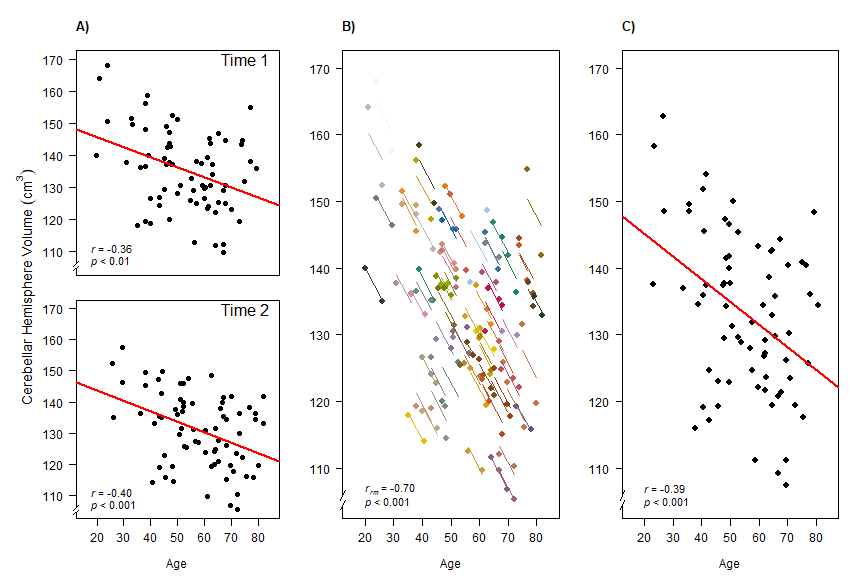#Figure 5. Comparison of rmcorr and simple regression/correlation results for age and brain structure volume data.
#Each dot represents one of two separate observations of age and CBH for a participant. (A) #Separate simple
#regressions/correlations by time: each observation is treated as independent, represented by shading all the data
#points black. The red line is the fit of the simple regression/correlation. (B) Rmcorr: observations from the same
#participant are given the same color, with corresponding lines to show the rmcorr fit for each participant. (C)
#Simple regression/correlation: averaged by participant. Note that the effect size is greater (stronger negative
#relationship) using rmcorr (B) than with either use of simple regression models (A) and (C). This figure was
#created using data from Raz et al. (2005).
#dev.copy2eps(file="plots/Figure5_Volume_Age.eps", width = 9, height = 6)
#dev.copy(pdf, file="plots/Figure5_Volume_Age.pdf", height = 6, width = 6)
#dev.off()

### 6. Visual search rmcorr and simple reg/cor results and Figure 6

#### rmcorr and simple reg results

#a - rmcorr
vissearch.rmc <- rmcorr(participant = sub, measure1 = rt, measure2 = acc, dataset = gilden2010)
#> Warning in rmcorr(participant = sub, measure1 = rt, measure2 = acc, dataset = gilden2010):
#> 'sub' coerced into a factor
print(vissearch.rmc)
#>
#> Repeated measures correlation
#>
#> r
#> -0.406097
#>
#> degrees of freedom
#> 32
#>
#> p-value
#> 0.01716871
#>
#> 95% confidence interval
#> -0.6611673 -0.06687244

#b - averaged data
gildenMeans <- aggregate(gilden2010[,3:4], by = list(gilden2010$sub), mean) avg.lm <- lm(acc ~ rt, data = gildenMeans) print(avg.lm) #> #> Call: #> lm(formula = acc ~ rt, data = gildenMeans) #> #> Coefficients: #> (Intercept) rt #> 0.8132 0.1777 b.rtest <- cor.test(gildenMeans$rt, gildenMeans$acc) print(b.rtest) #> #> Pearson's product-moment correlation #> #> data: gildenMeans$rt and gildenMeans$acc #> t = 2.1966, df = 9, p-value = 0.05565 #> alternative hypothesis: true correlation is not equal to 0 #> 95 percent confidence interval: #> -0.01409542 0.87910346 #> sample estimates: #> cor #> 0.5907749 #c - aggregated data (overfit, incorrectly treated as independent participants/observations) agg.lm <- lm(acc ~ rt, data = gilden2010) print(agg.lm) #> #> Call: #> lm(formula = acc ~ rt, data = gilden2010) #> #> Coefficients: #> (Intercept) rt #> 0.8612 0.1111 c.rtest <- cor.test(gilden2010$rt, gilden2010$acc) print(c.rtest) #> #> Pearson's product-moment correlation #> #> data: gilden2010$rt and gilden2010$acc #> t = 2.6401, df = 42, p-value = 0.01158 #> alternative hypothesis: true correlation is not equal to 0 #> 95 percent confidence interval: #> 0.09053513 0.60625185 #> sample estimates: #> cor #> 0.3772751 par(mfrow=c(1,3), mar=c(5,4.6,4,0.5), mgp=c(3.2,0.8,0), oma = c(0, 0, 0, 0), las = 1, cex.axis = 1.2, cex.sub = 1.1, cex.lab = 1.2) #, cex.axis = 1.10, cex.sub = 1.10, cex.lab = 1.15) plot(vissearch.rmc, overall = F, xlab = "Response Time (seconds)", ylab = "Accuracy", cex = 1.2, ylim = c(.79, 1), xlim = c(0.45, .95)) axis.break(axis = 1, style = "slash") axis.break(axis = 2, style = "slash") #example of rounding and pvals.fct inside text() text(0.95,0.8, adj = 1, bquote(italic(r[rm])~"="~.(round(vissearch.rmc$r, digits = 2))), cex = 1.2)
text(0.95,0.7925, adj = 1, bquote(italic('p')~"<"~.(pvals.fct(vissearch.rmc$p))), cex = 1.2) title("A)", adj = 0) plot(acc~rt, data = gildenMeans, cex = 1.2, pch = 16, ylim = c(.79, 1), xlim = c(0.45, .95), xlab = "Response Time (seconds)", ylab = "") abline(avg.lm, col = "red", lwd = 2) axis.break(axis = 1, style = "slash") axis.break(axis = 2, style = "slash") text(0.95,0.8, adj = 1, bquote(italic('r')~"="~ .(round(b.rtest$estimate, digits = 2))), cex = 1.2)
text(0.95,0.7925, adj = 1, bquote(italic('p')~"="~.(pvals.fct(b.rtest$p.value))), cex = 1.2) #text(.95,.8,paste('r =', round(b.rtest$est,2),'\np =', round(b.rtest$p.value,2)), adj = 1) title("B)", adj = 0) plot(acc~rt, data = gilden2010, xlab = "Response Time (seconds)", ylab = "", cex = 1.2, pch = 16, ylim = c(.79, 1), xlim = c(0.45, .95)) abline(agg.lm, col = "red", lwd = 2) axis.break(axis = 1, style = "slash") axis.break(axis = 2, style = "slash") text(0.95,0.8, adj = 1, bquote(italic('r')~"="~ .(round(c.rtest$estimate, digits = 2))), cex = 1.2)
text(0.95,0.7925, adj = 1, bquote(italic('p')~"<"~.(pvals.fct(c.rtest$p.value))), cex = 1.2) title("C)", adj = 0)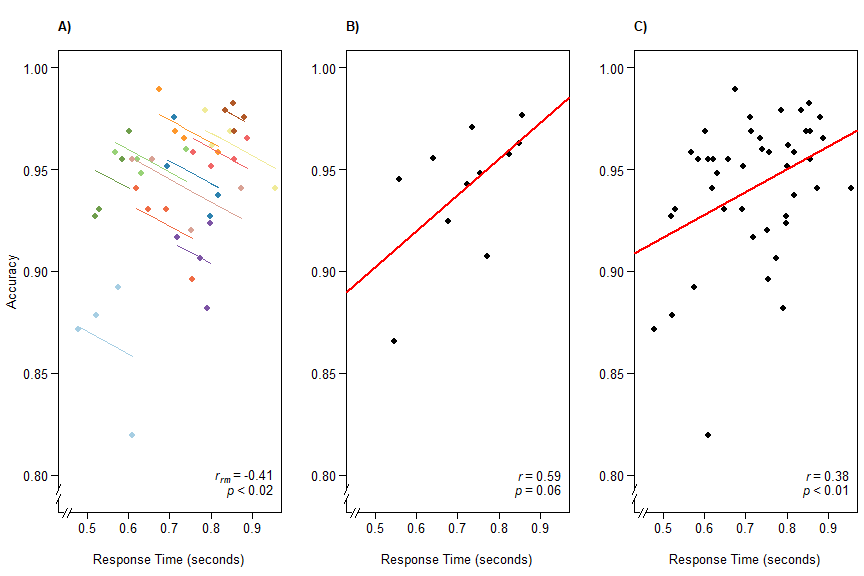#text(.95,.8,paste('r =', round(c.rtest$est,2),'\np =', round(c.rtest$p.value,2)), adj = 1) #Figure 6. The x-axis is reaction time (seconds) and the y-axis is accuracy in visual search. (A) Rmcorr: each dot represents #the average reaction time and accuracy for a block, color identifies participant, #and colored lines show rmcorr fits for #each participant. (B) Simple regression/correlation (averaged data): each dot represents a block, (improperly) treated as an #independent observation. The red line is #the fit to the simple regression/correlation. (C) Simple regression/correlation #(aggregated data): improperly treating each dot as independent. This figure was created using data from Gilden et al. (2010). #dev.copy2eps(file="plots/Figure6_Visual_Search.eps", width = 9, height = 6) #dev.copy(pdf, file="plots/Figure6_Visual_Search.pdf", height = 9, width = 6) #dev.off() ## Appendix C 1. Rmcorr and multilevel model with Raz et al. 2005 data brainvolage.rmc <- rmcorr(participant = Participant, measure1 = Age, measure2 = Volume, dataset = raz2005) #Null multilevel model: Random intercept and fixed slope null.vol <- lmer(Volume ~ Age + (1 | Participant), data = raz2005, REML = FALSE) #Model fit null.vol #> Linear mixed model fit by maximum likelihood ['lmerMod'] #> Formula: Volume ~ Age + (1 | Participant) #> Data: raz2005 #> AIC BIC logLik deviance df.resid #> 977.4705 989.3497 -484.7352 969.4705 140 #> Random effects: #> Groups Name Std.Dev. #> Participant (Intercept) 11.287 #> Residual 3.024 #> Number of obs: 144, groups: Participant, 72 #> Fixed Effects: #> (Intercept) Age #> 163.7090 -0.5533 #Parameter Confidence Intervals confint(null.vol) #> Computing profile confidence intervals ... #> 2.5 % 97.5 % #> .sig01 9.5208850 13.6036847 #> .sigma 2.5713387 3.6236647 #> (Intercept) 155.1479333 172.3796868 #> Age -0.7016833 -0.4056009 #Model fitted values and confidence intervals for each participant (L1 effects) set.seed(9999) L1.predict.raz <- predictInterval(null.vol, newdata = raz2005, n.sims = 1000) L1.predict.raz <- cbind(raz2005$Participant, L1.predict.raz)

theme_minimal =  theme_bw() +
theme(
legend.position="none",
axis.line.x = element_line(color="black", size = 0.9),
axis.line.y = element_line(color="black", size = 0.9),
axis.text.x = element_text(size = 12),
axis.text.y = element_text(size = 12),
axis.title.x = element_text(size = 12),
axis.title.y = element_text(size = 12)
)

#Create custom color palette
# Blues<-brewer.pal(9,"Blues")

ggplot(raz2005, aes(x = Age, y = Volume, group = Participant, color = Participant)) +
geom_line(aes(y = predict(null.vol)), linetype = 2) +
geom_line(aes(y = brainvolage.rmc$model$fitted.values), linetype = 1) +
geom_ribbon(aes(ymin = L1.predict.raz$lwr, ymax = L1.predict.raz$upr,
group = L1.predict.raz$raz2005$Participant,
linetype = NA), alpha = 0.07) +
theme_minimal +
labs(title = "Rmcorr and Random Intercept Multilevel Model:\n Raz et al. 2005 Data", x = "Age",
y = expression(Cerebellar~Hemisphere~Volume~(cm^{3}))) +
geom_point(aes(colour = Participant)) +
scale_colour_gradientn(colours=kelly(22)) + theme(plot.title = element_text(hjust = 0.5)) +
geom_abline(intercept = fixef(null.vol), slope = fixef(null.vol), colour = "black", size = 1, linetype = 2)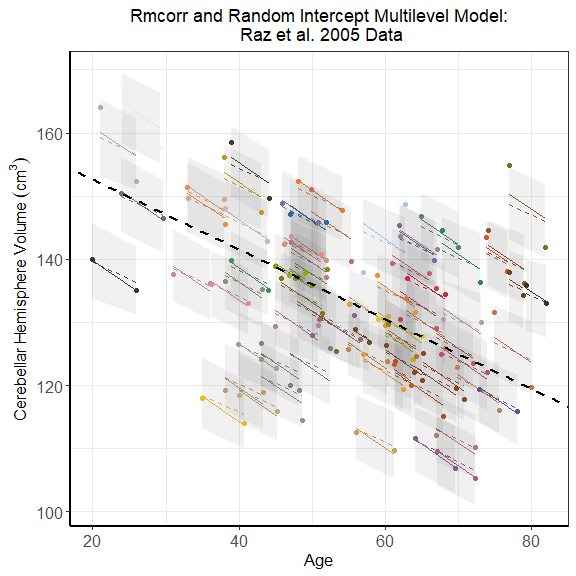#Appendix C, Figure 1: Dots are actual data values, with color indicating participant. Solid
#colored lines show the rmcorr model fit. The multilevel model fit is indicated by the dashed
#colored lines for Level 1 (participant) effects and the dashed black line for Level 2 (experiment)
#effects. The shaded areas are 95% confidence intervals for Level 1 effects. Note the models
#clearly overlap, despite the absence of confidence intervals for rmcorr.

#Converted to EPS file using Acrobat Pro b/c EPS doesn't support transparency
#ggsave(file = "plots/AppendixC_Figure1.pdf", width = 5.70 , height = 5.73, dpi = 300)
#dev.off()
1. Rmcorr and multilevel model with Gilden et al. 2010 data
vissearch.rmc <- rmcorr(participant = sub, measure1 = rt, measure2 = acc, dataset = gilden2010)

null.vis <- lmer(acc ~ rt + (1 | sub), data = gilden2010, REML = FALSE)

#Model 1: Random intercept + random slope for RT
model1.vis <- lmer(acc ~ rt + (1 + rt | sub), data = gilden2010, REML = FALSE)
#> boundary (singular) fit: see ?isSingular

#Model Comparison
#a) Chi-Square
anova(null.vis, model1.vis)
#> Data: gilden2010
#> Models:
#> null.vis: acc ~ rt + (1 | sub)
#> model1.vis: acc ~ rt + (1 + rt | sub)
#>            npar     AIC     BIC logLik deviance  Chisq Df Pr(>Chisq)
#> null.vis      4 -197.41 -190.27 102.70  -205.41
#> model1.vis    6 -193.46 -182.75 102.73  -205.46 0.0497  2     0.9754

#b) Evidence ratio using AIC
Models.vis<-list()
Models.vis<-c(null.vis, model1.vis)

if (requireNamespace("AICcmodavg", quietly = TRUE)){
ModelTable2<-aictab(Models.vis, modnames = c("null", "Model 1"))
ModelTable2
evidence(ModelTable2)
}
#>
#> Evidence ratio between models 'null' and 'Model 1':
#> 13.43

#Estimating and graphing null model
#Parameter Confidence Intervals
confint(null.vis)
#> Computing profile confidence intervals ...
#>                   2.5 %     97.5 %
#> .sig01       0.02138120 0.05796124
#> .sigma       0.01294851 0.02139924
#> (Intercept)  0.91815424 1.05718722
#> rt          -0.15404840 0.02955915

#Model fitted values and confidence intervals for each participant (L1 effects)
set.seed(9999)
L1.predict.gilden <- predictInterval(null.vis, newdata = gilden2010, n.sims = 1000)
L1.predict.gilden <- cbind(gilden2010$sub, L1.predict.gilden) theme_minimal = theme_bw() + theme( legend.position="none", axis.line.x = element_line(color="black", size = 0.9), axis.line.y = element_line(color="black", size = 0.9), axis.text.x = element_text(size = 12), axis.text.y = element_text(size = 12), axis.title.x = element_text(size = 12), axis.title.y = element_text(size = 12) ) #Create custom color palette Colors12<-brewer.pal(12,"Paired") ggplot(gilden2010, aes(x = rt, y = acc, group = sub, color = sub)) + geom_line(aes(y = predict(null.vis)), linetype = 2) + geom_line(aes(y = vissearch.rmc$model$fitted.values), linetype = 1) + geom_ribbon(aes(ymin = L1.predict.gilden$lwr,
ymax = L1.predict.gilden$upr, group = L1.predict.gilden$gilden2010$sub, linetype = NA), alpha = 0.07) + theme_minimal + theme(plot.title = element_text(hjust = 0.5)) + labs(title = "Rmcorr and Random Intercept Multilevel Model:\n Gilden et al. 2010 Data", x = "Response Time (Seconds)", y = "Accuracy") + geom_point(aes(colour = sub)) + scale_colour_gradientn(colours=Colors12) + geom_abline(intercept = fixef(null.vis), slope = fixef(null.vis), colour = "black", size = 1, linetype = 2) + scale_y_continuous(breaks=seq(0.80, 1.0, 0.05)) + scale_x_continuous(breaks=seq(0.50, 0.9, 0.1))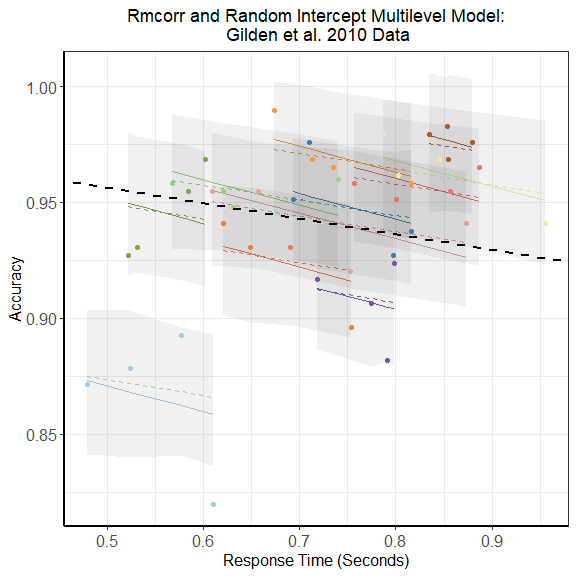#Converted to EPS file using Acrobat Pro b/c EPS doesn't support transparency #Appendix C, Figure 2: Dots are actual data values, with color indicating participant. Solid #colored lines show the rmcorr model fit. The multilevel model fit is indicated by the dashed #colored lines for Level 1 (participant) effects and the dashed black line for Level 2 (experiment) #effects. The shaded areas are 95% confidence intervals for Level 1 effects. Note the models #clearly overlap, despite the absence of confidence intervals for rmcorr. #ggsave(file = "plots/AppendixC_Figure2.pdf", width = 6.5 , height = 6.5, dpi = 300) #Estimating CIs: Convergence problems with model 1 confint(model1.vis) #> Computing profile confidence intervals ... #> 2.5 % 97.5 % #> .sig01 0.02197527 0.11293808 #> .sig02 -1.00000000 1.00000000 #> .sig03 0.00000000 Inf #> .sigma 0.01303108 0.02157782 #> (Intercept) 0.91458725 1.06378202 #> rt -0.15425292 0.03364490 warnings() set.seed(9999) predictInterval(model1.vis, newdata = gilden2010, n.sims = 1000) #> fit upr lwr #> 1 0.8663920 0.8960908 0.8352832 #> 2 0.8683327 0.8992494 0.8385416 #> 3 0.8707389 0.8997923 0.8393785 #> 4 0.8725921 0.9043416 0.8411908 #> 5 0.9429413 0.9732029 0.9159473 #> 6 0.9432319 0.9722811 0.9162444 #> 7 0.9500212 0.9787765 0.9220920 #> 8 0.9521220 0.9792167 0.9237837 #> 9 0.9481541 0.9752953 0.9207015 #> 10 0.9557798 0.9844996 0.9267692 #> 11 0.9559257 0.9853144 0.9268080 #> 12 0.9596370 0.9887583 0.9285575 #> 13 0.9443758 0.9730895 0.9144351 #> 14 0.9430301 0.9705867 0.9121712 #> 15 0.9481910 0.9777425 0.9189827 #> 16 0.9500669 0.9785099 0.9180516 #> 17 0.9546490 0.9828561 0.9234057 #> 18 0.9511326 0.9826628 0.9211205 #> 19 0.9583308 0.9867742 0.9280774 #> 20 0.9601212 0.9901520 0.9312060 #> 21 0.9227967 0.9488316 0.8935664 #> 22 0.9244902 0.9553428 0.8959148 #> 23 0.9277636 0.9569939 0.8986043 #> 24 0.9300477 0.9599092 0.9000850 #> 25 0.9625122 0.9928161 0.9347764 #> 26 0.9680937 0.9978023 0.9390449 #> 27 0.9706817 1.0010774 0.9416283 #> 28 0.9749069 1.0033890 0.9446229 #> 29 0.9337347 0.9613853 0.9051130 #> 30 0.9427611 0.9688831 0.9133651 #> 31 0.9495472 0.9767039 0.9189270 #> 32 0.9506709 0.9813415 0.9226078 #> 33 0.9092402 0.9374052 0.8801574 #> 34 0.9081569 0.9380428 0.8783812 #> 35 0.9101271 0.9393227 0.8784178 #> 36 0.9130451 0.9411345 0.8840515 #> 37 0.9530667 0.9841683 0.9233226 #> 38 0.9633384 0.9921144 0.9363187 #> 39 0.9616431 0.9909585 0.9335434 #> 40 0.9655959 0.9935503 0.9362402 #> 41 0.9739775 1.0029519 0.9440092 #> 42 0.9725509 1.0019449 0.9447089 #> 43 0.9767107 1.0060772 0.9455765 #> 44 0.9756523 1.0049782 0.9452859 warnings() ### Diagnostic Plot: Rmcorr and straight lines between points (not in paper) brainvolage.rmc <- rmcorr(participant = Participant, measure1 = Age, measure2 = Volume, dataset = raz2005) #> Warning in rmcorr(participant = Participant, measure1 = Age, measure2 = Volume, : #> 'Participant' coerced into a factor print(brainvolage.rmc) #> #> Repeated measures correlation #> #> r #> -0.7044077 #> #> degrees of freedom #> 71 #> #> p-value #> 3.561007e-12 #> #> 95% confidence interval #> -0.8053581 -0.5637514 theme_minimal = theme_bw() + theme( legend.position="none", axis.line.x = element_line(color="black", size = 0.9), axis.line.y = element_line(color="black", size = 0.9), axis.text.x = element_text(size = 12), axis.text.y = element_text(size = 12), axis.title.x = element_text(size = 12), axis.title.y = element_text(size = 12) ) ggplot(raz2005, aes(x = Age, y = Volume, group = Participant, color = Participant)) + geom_line(aes(y = brainvolage.rmc$model\$fitted.values), linetype = 1) +
theme_minimal +
labs(title = "Rmcorr and Diagnostic Lines:\n Raz et al. 2005 Data", x = "Age",
y = expression(Cerebellar~Hemisphere~Volume~(cm^{3}))) +
geom_point(aes(colour = Participant)) +
scale_colour_gradientn(colours=kelly(22)) + theme(plot.title = element_text(hjust = 0.5)) + geom_line(linetype = 3)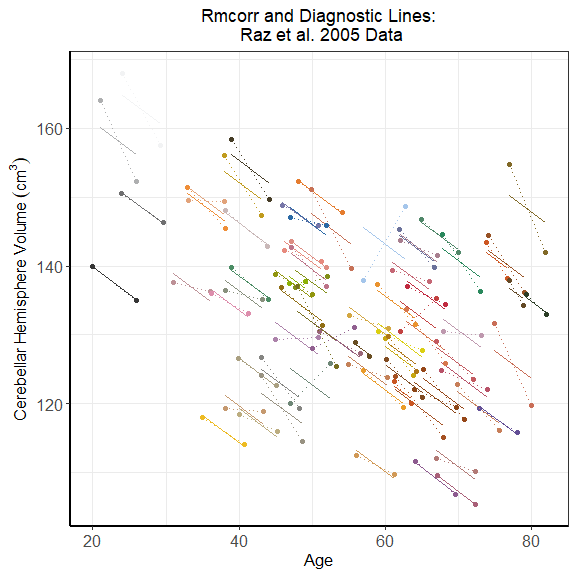#ggsave(file = "plots/Figure_rmcorr_diagnostic.pdf", width = 5.70 , height = 5.73, dpi = 300)
#ggsave(file = "plots/Figure_rmcorr_diagnostic.eps", width = 5.70 , height = 5.73, dpi = 300)
#dev.off()